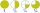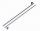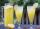#### Number of problems found: 1447

• Two dividedTwo divided by nine tenths.
• EquatiomSolve equation with negatives: X/(-5) + 2 = -9
• Negative in equation2x + 3 + 7x = – 24, what is the value of x?
• If-then equationIf 5x - 17 = -x + 7, then x =
• EquationFind x: x + 1/2 = 1/3
• Equation?
• RoundingThe following numbers round to the thousandth:
• Angles 1It is true neighboring angles have not common arm?
• A quotientWhat is the quotient of 3/10 divided by 2/4 as a fraction?
• Cupcakes 2Susi has 25 cupcakes. She gives 4/5. How much does she have left?
• Eq1Solve equation: 4(a-3)=3(2a-5)
• SimplifySimplify the following problem and express as a decimal: 5.68-[5-(2.69+5.65-3.89) /0.5]
• PlaneOn how many parts divide plane 6 parallels?
• MilimetersHow many millimeters is 1/4 meters?How many 1/4 cup servings are in 2 and 1/3 cups of lemonade?
• The shopThe shop has 3 hectoliters of water. How many liter bottles is it?
• Round it0.728 round to units, tenths, hundredths.What is 4 1/2+2/7-213/14?3 3/4 + 2 3/5 + 5 1/2 Show your solution.How many hours are in 3 2/3 days?